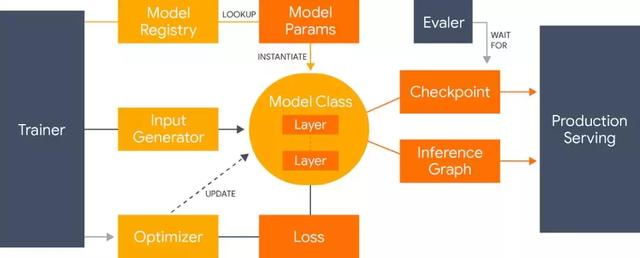Lingvo 是世界语（Esperanto）中的一个单词，它表示「语言」的意思。这一命名展示了 Lingvo 框架的根源：它是由 TensorFlow 开发的通用深度学习框架，它重点关注自然语言处理相关的序列建模方法，包括机器翻译、语音识别和语音合成等。

Lingvo 主要支持大量研究团体在一个共享代码库中从事语音和自然语言处理相关问题的研究。它的设计原则如下：

• 单个代码块应该精细且模块化，它们会使用相同的接口，同时也容易扩展；
• 实验应该是共享的、可比较的、可复现的、可理解的和正确的；
• 性能应该可以高效地扩展到生产规模的数据集，或拥有数百个加速器的分布式训练系统；
• 当模型从研究转向产品时应该尽可能共享代码。Lingvo 是在考虑协作研究的基础上构建的，它主要通过在不同任务之间共享公共层的实现，从而提升代码的复用程度。此外，所有层都实现了相同的公共接口，并以相同的方式布局代码结构。这不仅会产生更简洁和易读的代码，同时其它任务上的改良也可以便捷地应用到我们的任务上。实现这种一致性代码确实会有更多的成本，例如更加规范和模板化的代码。但是 Lingvo 也在尝试减少这种成本，以确保更快地迭代研究成果。

p = model.AsrModel.Params()
p.name = ‘librispeech’
# Initialize encoder params.
ep = p.encoder
# Data consists 240 dimensional frames (80 x 3 frames), which we
# LibrispeechCommonAsrInputParams.
ep.input_shape = [None, None, 80, 1]
ep.lstm_cell_size = 1024
ep.num_lstm_layers = 4
ep.conv_filter_shapes = [(3, 3, 1, 32), (3, 3, 32, 32)]
ep.conv_filter_strides = [(2, 2), (2, 2)]
ep.cnn_tpl.params_init = py_utils.WeightInit.Gaussian(0.001)
# Disable conv LSTM layers.
ep.num_conv_lstm_layers = 0
# Initialize decoder params.
dp = p.decoder
dp.rnn_cell_dim = 1024
dp.rnn_layers = 2
dp.source_dim = 2048
# Use functional while based unrolling.
dp.use_while_loop_based_unrolling = False
tp = p.train
tp.learning_rate = 2.5e-4
tp.lr_schedule = lr_schedule.ContinuousLearningRateSchedule.Params().Set(
start_step=50000, half_life_steps=100000, min=0.01)
# Setting p.eval.samples_per_summary to a large value ensures that dev,
# devother, test, testother are evaluated completely (since num_samples for
# each of these sets is less than 5000), while train summaries will be
# computed on 5000 examples.
p.eval.samples_per_summary = 5000
p.eval.decoder_samples_per_summary = 0
# Use variational weight noise to prevent overfitting.
p.vn.global_vn = True
p.train.vn_std = 0.075
p.train.vn_start_step = 20000
return p